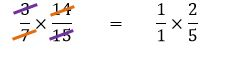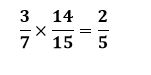# How to multiply fractions

Want to find out how to multiply fractions? We've provided a step-by-step guide of how to multiply fractions to teach you everything you need to know.## Step 1: Look for common factors shared between any of the numerators and the denominators.

Given two fractions are being multiplied, look for common factors shared between any of the numerators and the denominators. (If working with mixed fraction, change the fraction to an improper fraction first).

Let’s say if we have 2/3×27/44

## Step 2: Multiply the remaining factors

Multiply the remaining factors to find the product of the fractions.

## Examples of how to multiply fractions

Q1) What is the result if we multiply 3/7 by 14/15?

Look for common factors shared by numerators and denominatorsMultiply the remaining factors to simplify the product.#### Join today

The fastest way to practice

Unlock our complete testing platform and improve faster that ever.